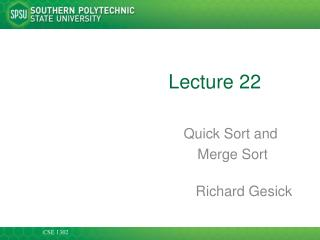DownloadDownload PresentationLecture 22

# Lecture 22

Download Presentation## Lecture 22

- - - - - - - - - - - - - - - - - - - - - - - - - - - E N D - - - - - - - - - - - - - - - - - - - - - - - - - - -
##### Presentation Transcript

1. Lecture 22 Quick Sort and Merge Sort Richard Gesick

2. Merge Sort • Sorts an array by • Cutting the array in half • Recursively sorting each half • Merging the sorted halves • Dramatically faster than the selection sort

3. Merge Sort

4. The key part of the MergeSort public void sort() { if (a.length <= 1) return; int[] first = new int[a.length / 2]; int[] second = new int[a.length - first.length]; System.Array.Copy(a, 0, first, 0, first.length); System.Array.Copy(a, first.length, second, 0, second.length); MergeSorterfirstSorter = new MergeSorter(first); MergeSortersecondSorter = new MergeSorter(second); firstSorter.sort(); secondSorter.sort(); merge(first, second); }

5. Merge Sort Vs Selection Sort • Selection sort is an O( n2 ) algorithm • Merge sort is an O( nlog(n) ) algorithm • The nlog(n) function grows much more slowly than n2

6. Merge Sort Example • Divide an array in half and sort each half, just working with the left half for now

7. Merge Sort Example • Then merge the sorted arrays

8. Merge Sort Example • Then the final Merge of the two sorted arrays into a single sorted array

9. The Sort public void sort () { if (a.Length <= 1) return; int[] first = new int[a.Length / 2]; int[] second = new int[a.Length - first.Length]; System.Array.Copy (a, 0, first, 0, first.Length); System.Array.Copy (a, first.Length, second, 0, second.Length); MergeSorterfirstSorter = new MergeSorter (first); MergeSortersecondSorter = new MergeSorter (second); firstSorter.sort (); secondSorter.sort (); merge (first, second); }

10. The Merge private void merge (int [] first, int [] second) { int iFirst = 0; // next element to consider in the first range int iSecond = 0; // next element to consider in the second range int j = 0; // next open position in a // as long as neither iFirst nor iSecond past the end, move // the smaller element into temp

11. The Merge while (iFirst < first.Length && iSecond < second.Length) { if (first [iFirst] < second [iSecond]) { a [j] = first [iFirst]; iFirst++; } else { a [j] = second [iSecond]; iSecond++; } j++; } System.Array.Copy(first, iFirst, a, j, first.Length - iFirst); System.Array.Copy(second, iSecond, a, j, second.Length - iSecond); }

12. Analyzing the Merge Sort Algorithm

13. Merge Sort Timing vs. Selection Sort Figure 2:Merge Sort Timing (blue) versus Selection Sort (red)

14. Merge Sort Vs Selection Sort Selection sort is an O(n2) algorithm Merge sort is an O(nlog(n)) algorithm The nlog(n) function grows much more slowly than n2

15. The Quick Sort • the quick sort is one of the fastest sorting processes available. • it uses a recursive, divide and conquer strategy.

16. the theory • the basic idea is to divide the list into two parts, based upon a point called the pivot, which is in the middle of the list{(index of first + index of last) / 2} • at the end of the process, one part will contain all the elements less than the pivot and the other part will contain all the elements larger than the pivot.

17. the process • array a has 11 elements a to a • 14 3 2 11 5 8 0 2 9 4 20 • 8 is the pivot • we assign a pointer called left arrow to a, the smallest index • and a pointer called right arrow to a, the largest index

18. more process • left pivot right • 14 3 2 11 5 8 0 2 9 4 20 • now we will start moving our arrows until we find values that should be exchanged. • starting with the right arrow, it is moved until we find a value less than or equal to the pivot. Then we move the left arrow until we find a value greater than or equal to the pivot. Once this occurs, we exchange values.

19. even more process (a) • pivot • 14 3 2 11 5 8 0 2 9 4 20 • left right • exchange the values and our new array is • pivot • 4 3 2 11 5 8 0 2 9 14 20 • left right • now start moving the arrows again • pivot • 4 3 2 11 5 8 0 2 9 14 20 • left right

20. even more process (b) • left pivot right • 4 3 2 2 5 8 0 11 9 14 20 • now the process will stop when the left arrow> right arrow • since it’s not true yet, continue on • pivot • 4 3 2 2 5 8 0 11 9 14 20 • left right • the left arrow stops on 8(the pivot) because we are looking for values greater than or equal to the pivot and now we swap again

21. even more process (c) • pivot • 4 3 2 2 5 0 8 11 9 14 20 • left right • left arrow is still less than right so continue the process • pivot • 4 3 2 2 5 0 8 11 9 14 20 • right left • the first subdivision is now complete and the 2 sublists can now be sorted using the same function

22. key parts of the QuickSort • public void quicksort( list_type list, int left, int right) • { • left_arrow=left; • right_arrow=right; • pivot=list[(left+right)/2]; • //. . . the recursive calls • if (left<right_arrow) quicksort(list, left, right_arrow); • if (left_arrow<right) quicksort(list, left_arrow,right);

23. QuickSort Analysis • like the merge sort, the quick sort ends up with a Big O of O(n log n)